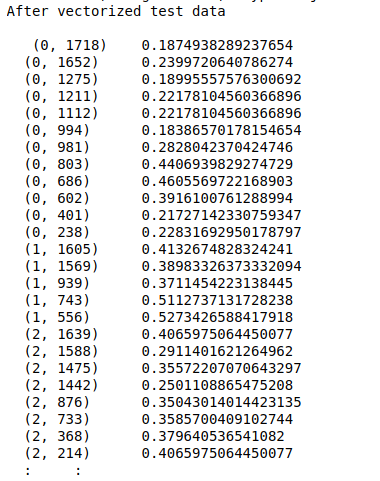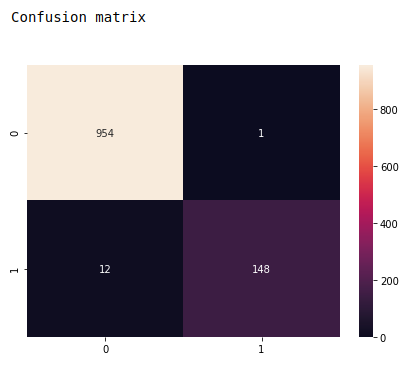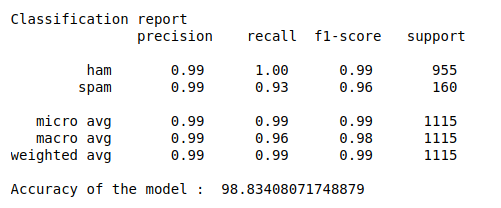• #5, First Floor, 4th Street Dr. Subbarayan Nagar Kodambakkam, Chennai-600 024 Landmark : Samiyar Madam
• pro@slogix.in
• +91- 81240 01111

### How to build spam detector using Multi Layer Perceptron in python?

###### Description

To build spam detector using MLP classifier in python

###### Input

Spam detection data set.

###### Output

Confusion matrix
Classification report
Accuracy score
Precision and recall

#### process:

Define the independent and dependent variable.

Convert the test data in to vector suing TF-IDF vectorizer..

Build the MLP classifier..

Initiate activation and optimizer functions according to the problem.

Fit the training set into the model.

Predict the test results using MLP classifier.

Calculate the accuracy, precision and recall.

###### Sample Code

#import necessary libraries
import warnings
warnings.filterwarnings(“ignore”)
import pandas as pd
import numpy as np
import seaborn as sns
import matplotlib.pyplot as plt
from sklearn.preprocessing import LabelEncoder
from sklearn.feature_extraction.text import TfidfVectorizer
from sklearn.model_selection import train_test_split
from sklearn import metrics
from sklearn.neural_network import MLPClassifier

names = [‘class’,’text’]

#make as a data frame
df = pd.DataFrame(data)

#Checking missing values
print(“Checking missing values\n”)
print(df.isnull().sum())

#variable selection
X = df[‘text’]
y = df[‘class’]

#Split train and test data
X_train, X_test, y_train, y_test = train_test_split(X, y, test_size=0.2, random_state=0)

#pre-processing
#Initialize the TF-IDF vectorizer
tfidf = TfidfVectorizer(sublinear_tf=True, min_df=5, norm=’l2′, encoding=’latin-1′, ngram_range=(1, 2),
stop_words=’english’)

#transform independent variable using TF-IDF vectorizer
X_train_tfidf = tfidf.fit_transform(X_train)
X_test_tfidf = tfidf.transform(X_test)

#Training and testing data
print(“\n”)
print(“After vectorized train data\n\n”,X_train_tfidf,”\n\n”,y_train)
print(“After vectorized test data\n\n”,X_test_tfidf)

#Neural network model

#fit the model
clf.fit(X_train_tfidf, y_train)

# Predicting the Test set results
y_pred = clf.predict(X_test_tfidf)

# Creating the Confusion Matrix
from sklearn.metrics import confusion_matrix
cm = confusion_matrix(y_test, y_pred)

print(“\n”,”Confusion matrix\n”)
fig, ax = plt.subplots(figsize=(7,5))
sns.heatmap(cm, annot=True, fmt=’d’)
plt.show()

print(“Classification report\n”,metrics.classification_report(y_test, y_pred))
print(“Accuracy of the model : “,metrics.accuracy_score(y_test, y_pred)*100)

###### Screenshots